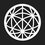# A nice problem!

I submitted a problem to brilliant which was unfortunately rejected. I thought it would be nice to share it with all of you. Here it is :  $\small 7$-digit numbers (without repetition of digits) are formed using the digits $\small \{1,2,3,4,5,6,7 \}$. Let $\small S$ be the set of all such numbers. How many pairs of $\small (a,b) \in S$ exist such that $b$ is a multiple of $a$ given that $\small a \neq b$7 years ago

This discussion board is a place to discuss our Daily Challenges and the math and science related to those challenges. Explanations are more than just a solution — they should explain the steps and thinking strategies that you used to obtain the solution. Comments should further the discussion of math and science.

When posting on Brilliant:

• Use the emojis to react to an explanation, whether you're congratulating a job well done , or just really confused .
• Ask specific questions about the challenge or the steps in somebody's explanation. Well-posed questions can add a lot to the discussion, but posting "I don't understand!" doesn't help anyone.
• Try to contribute something new to the discussion, whether it is an extension, generalization or other idea related to the challenge.

MarkdownAppears as
*italics* or _italics_ italics
**bold** or __bold__ bold
- bulleted- list
• bulleted
• list
1. numbered2. list
1. numbered
2. list
Note: you must add a full line of space before and after lists for them to show up correctly
paragraph 1paragraph 2

paragraph 1

paragraph 2

[example link](https://brilliant.org)example link
> This is a quote
This is a quote
    # I indented these lines
# 4 spaces, and now they show
# up as a code block.

print "hello world"
# I indented these lines
# 4 spaces, and now they show
# up as a code block.

print "hello world"
MathAppears as
Remember to wrap math in $$ ... $$ or $ ... $ to ensure proper formatting.
2 \times 3 $2 \times 3$
2^{34} $2^{34}$
a_{i-1} $a_{i-1}$
\frac{2}{3} $\frac{2}{3}$
\sqrt{2} $\sqrt{2}$
\sum_{i=1}^3 $\sum_{i=1}^3$
\sin \theta $\sin \theta$
\boxed{123} $\boxed{123}$

Sort by:

This is basically a case checking:

We can have the multiples in the range $[2,7]$

When the multiple is $2$ it is not possible, because when you multiply $4$[in the number] and $2$ , the resulting digit can be either $8$ or $9$ [The greatest carry over can be only $1$ ].

When the multiple is $3$, the digit $6$ when multiplied by $3$ can only give either $8,9,0$ which is again not possible.

When the multiple is $4$, the digit $2$ in the number when multiplied will produce the corresponding digit $8,9,0$ since highest carry over is $2$. This is also not possible.

When it is $5$: Now notice that the $1 \times 5=5$, $3 \times 5$ gives us another digit $5$ in the multiplication of the the $7$ digit number. Also $7 \times 5$ gives us the same.Also $5 \times 5$ gives us another $5$ to deal with. Now out of these we need one of them to stay $5$ and other to either become $6$ or $7$. But, the other if it is distinct from other numbers will exceed $7$. So no numbers here either.

When it is $6$:

$1 \times 6$ and $6 \times 6$ gives us $6$.

So to preserve non-repetition, on one has to be $6$ and the other $7$.

First note the first digit of $a$ has to be $1$ or our multiple will be out of the domain. This implies that the last digit has to be $6$ [carry over concept].

So, if a pair exists in this case, then $a$ has to have $1$ in the first place and $6$ in the last.

Now the second last digit that can survive[stay in our range] the carry over $3$ derived from the last digit is either $2$ or $3$. If it is $2$ then the number next to $1$ can only be $3$ since $3 \times 6$ will gives us a carry over $1$. But, wait the other numbers left [$4,5,7$ ] will ensure this does not happen.

So, the number before $6$ can be $3$ and the number after $1$ has to be $2$.

The digit before $3$ cannot be $7$ because the numbers to be placed before $7$ are only $5$ or $4$. If it is $5$ we have a repetition of the digit $4$ and if the digit before 7 is 4, then we have an $8$ in the resulting digit. So, $7$ must come after $2$. Great, this is not possible since that will make our 2nd digit of $b$ as $6$, but $6$ is already the last digit.

Now when the multiple is $7$ : It is obviously not possible since the first digit will be greater than $8$ no matter how you place the digits.

There might be some parts that are incoherent, and I might have easily missed something, in any case let me know if the answer to the question is not $0$.

- 7 years ago

The answer is correct and I like the solution as well!

- 7 years ago

Every number is of the form 9x+1, and since the max we can multiply $a$ is by 7, there are no such numbers. Nice problem.

- 7 years ago

Yeah I had the same solution. All numbers are $1$ mod $9$. And $\large \frac{b}{a} \small < 7$. So basically there cannot exist another number that is $1$ mod $9$

- 7 years ago

Did they give you a reason for rejection?

- 7 years ago

They said it did not fit in any of the problem categories.

- 7 years ago

I see. Thanks.

- 7 years ago